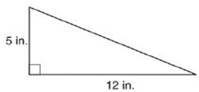Chapter 8.2, Problem 1E### Elementary Geometry for College St...

6th Edition
Daniel C. Alexander + 1 other
ISBN: 9781285195698

#### Solutions

Chapter
Section### Elementary Geometry for College St...

6th Edition
Daniel C. Alexander + 1 other
ISBN: 9781285195698
Textbook Problem
78 views

# In Exercises 1 to 8, find the perimeter of each polygon.To determine

To Find:

Perimeter of the triangle provided.

Explanation

Formula Used:

The perimeter of a polygon is the sum of the lengths of all sides of the polygon.

Pythagorean theorem for the right angle triangle ABC for the hypotenuse AC,

AC2=AB2+BC2.

Calculation:

Let the vertices of the provided triangle be A, B and C with the hypotenuse AC.

It is observed from the provided triangle that AB=5 in. and BC=12 in.

Applying the Pythagorean theorem,

AC2=AB2+BC2

### Still sussing out bartleby?

Check out a sample textbook solution.

See a sample solution

#### The Solution to Your Study Problems

Bartleby provides explanations to thousands of textbook problems written by our experts, many with advanced degrees!

Get Started

#### Find more solutions based on key concepts